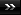View Single Post01-23-2010, 04:08 PM   #886
abraxasinas
_

Join Date: Dec 2009
Location: Queanbeyan/Canberra; NSW, Australia
Posts: 635Re: Thuban Q&A: (warning longer than normal posts here)

[QUOTE=Initiate;227129]
Quote:
 Originally Posted by abraxasinasThe 'big picture' encompasses your cosmic ID of being within Spacetime simultaneously with being without it. Initiate asked a very good question. How can something exist in nothing? Knowing a little mathematics, envisage an expanding series of the form: 0/1+1/2+2/3+3/4+...+n/(n+1)+...99999/1000000+...always getting bigger as a fraction or a decimal but never actually reaching the limit of 1/1=1. This way, the Mirror of the Infinity in 0/0=∞/∞=1, because 0=0 and ∞=∞ to define the inversion of 1/1=1 in the undefinability of 1/0≡∞ ↔ ∞.0≡1 and limited in the mathematical symbolisation in the physicalisations of limits in 1/∞→0 and 1/0→∞ as the reciprocities of each other by 0/1≡0 and ∞/1≡∞. All of Creation = 1 The Void = 0 The Eternity = 1/0=Infinity meaning that 0xInfinity=1! (not in the physicalised limit but by definition) if we take the algebra a / b = c then c * b = a. I understand this to be a fundemental law of mathematics. Now, in order for us to calculate c, when a = 1 and b = ∞ then 1/∞ = c and c * ∞ = 1 what is c? c can not equal 0 because if 1/∞ = 0 (c) then 0 (c) * ∞ = 0 and not 1 c must be a number infinitely close to 0 but not equal to 0. so 0 * ∞ doesn't equal 1 The Eternity = 1/0=Infinity meaning that 0xInfinity=1 is false near 0 (c) * ∞ can = 1 but c can not be defined on its own. Who told you that! The key point is NEAR, neighbourhood of points, Calculus LIMITS in differential Geometry. This is physical not meaphysical. Using IDENTITY in 0/0=∞/∞=1, because 0=0 and ∞=∞ to define the inversion of 1/1=1 in the undefinability of 1/0≡∞ ↔∞.0≡1 and limited in the mathematical symbolisation in the physicalisations of limits in 1/∞→0 and 1/0→∞ as the reciprocities of each other by 0/1≡0 and ∞/1≡∞. So USING ABOVE the question of How many Zeros add up to 1 is answered as Infinity. am I missing something?

Yes, NEAR 0 c* the Mathematics CAN BE refined in Metaphysical terms.

The MetaMathematics of Divisibility
Consider the maximum conditions of a system as being the inverse of the minimum conditions. This in a nutshell is Modular Duality as discussed in QR.

Allow this LINEAR scale from minimum to maximum to RECIRCULARISE itself, so reflecting the initialising process of something NOT describable in 'measurable' terms to THEN become measurable. This is the Mathematics of 0=1=∞=Infinity as some metaphysical ultimate Identity, which 'bounds' all of the mensuration physics in principles of mathematics.

The major principle here is that DIVISION DOES NOT EXIST.
So one cannot divide 0 nor 1 nor ∞=Infinity.
Then the Identities 0=0 and 1=1 and ∞=∞ form the SELFINTERACTION of the UNDIVIDED system.
The link to DIVISION and partitioning of the UNITY in THREE (Circular closure symbolised by cipher '0' ; its unfolding symbolised by cipher '1' and its multiplication symbolised by cipher '8' as 'two circles' moebian-connected in inventing 3D from 2D and 1D and 0D*) then are the ASYMPTOTES or LIMITS of the ratios 0/0=1/1=∞/∞.

{That is 3D-Volume as locus of a surface-point around a centre is contained within a 2-Sphere, whose 2D-Surface-Area is the locus of a circle as a 1-Sphere, rotated about the centre generating the sphere say. Defining this centre as midway between two endpoints then defines the 0-Sphere as 1D-Line from the 0D-Centre}.

This LIMIT must be 1, because only IF it is 1 do the Identities 0=0 and 1=1 and ∞=∞ hold true in the mapped system of the MetaMathematics or OmniMathematics becoming Mathematics, where only the Division of 1/1=1 is allowed by definition.
One could then define 0/0=1=1/1=∞/∞; 0/1=0 and 0/∞=02 for 0=∞.02 in 1/∞=0 and 1/0=∞ and ∞/0=∞2 for 1/0=∞.
Those definitions of OmniMathematics then become the limits of Mathematics by MetaMathematics.

The Division-Transformation dij=(0,1,∞) then can be expressed in the square matrix:

|1 Infinity Infinity2|
|0 1 Infinity | = D with Determinant detD,
|0^2 0 1 |
where detD=1(1^2-0.Infinity)-Infinity(0.1-0^2.Infinity)+Infinity2(0.0-1.0^2)
=1^3-1.0.Infinity-Infinity.0.1+(Infinity.0)2
=1^3-2.1^2+1^2=0

Thus matrix D is singular and has no inverse, all cofactors being also 0.
Matrix D is however symmetric in its transpose (exchanging rows i with columns j) for detD^T=0.

The Feynman Path so sums both negative and positive integers as:
-n......-3...-2...-1...0...1...2...3......n =T(n)=n(n+1) in absolute value to double the infinities as the entropy reversal of lightpath x=c.t=(-c)(-t) in the Möbius Property of the 4 worlds.

For the elementary Euler-Identy encompassing all dimensions in the Fibonacci-Roots X and Y then:
e^iπ =XY=X+Y= i² =cos(π)+isin(π) = -1

Cardinality Aleph-Null: lim[n->∞]{T(n)}=∞
Cardinality Aleph-All: lim [n->X]{T(n)}=1

Cantor Cardinality Aleph-Null is thus Unitised in Aleph-All, counting infinities as if they were integers of the Feynman Path.

AA

Last edited by abraxasinas; 01-23-2010 at 04:16 PM.